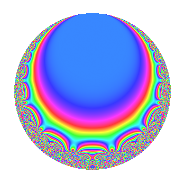# Properties

 Label 14.5.bLevel 14 Weight 5 Character orbit b Rep. character $$\chi_{14}(13,\cdot)$$ Character field $$\Q$$ Dimension 4 Newforms 1 Sturm bound 10 Trace bound 0

# Related objects

## Defining parameters

 Level: $$N$$ = $$14 = 2 \cdot 7$$ Weight: $$k$$ = $$5$$ Character orbit: $$[\chi]$$ = 14.b (of order $$2$$ and degree $$1$$) Character conductor: $$\operatorname{cond}(\chi)$$ = $$7$$ Character field: $$\Q$$ Newforms: $$1$$ Sturm bound: $$10$$ Trace bound: $$0$$

## Dimensions

The following table gives the dimensions of various subspaces of $$M_{5}(14, [\chi])$$.

Total New Old
Modular forms 10 4 6
Cusp forms 6 4 2
Eisenstein series 4 0 4

## Trace form

 $$4q$$ $$\mathstrut +\mathstrut 32q^{4}$$ $$\mathstrut -\mathstrut 76q^{7}$$ $$\mathstrut -\mathstrut 252q^{9}$$ $$\mathstrut +\mathstrut O(q^{10})$$ $$4q$$ $$\mathstrut +\mathstrut 32q^{4}$$ $$\mathstrut -\mathstrut 76q^{7}$$ $$\mathstrut -\mathstrut 252q^{9}$$ $$\mathstrut +\mathstrut 360q^{11}$$ $$\mathstrut -\mathstrut 288q^{14}$$ $$\mathstrut +\mathstrut 384q^{15}$$ $$\mathstrut +\mathstrut 256q^{16}$$ $$\mathstrut +\mathstrut 192q^{18}$$ $$\mathstrut +\mathstrut 768q^{21}$$ $$\mathstrut -\mathstrut 1152q^{22}$$ $$\mathstrut -\mathstrut 792q^{23}$$ $$\mathstrut -\mathstrut 2300q^{25}$$ $$\mathstrut -\mathstrut 608q^{28}$$ $$\mathstrut +\mathstrut 1224q^{29}$$ $$\mathstrut +\mathstrut 4416q^{30}$$ $$\mathstrut +\mathstrut 4032q^{35}$$ $$\mathstrut -\mathstrut 2016q^{36}$$ $$\mathstrut -\mathstrut 3896q^{37}$$ $$\mathstrut -\mathstrut 768q^{39}$$ $$\mathstrut -\mathstrut 4800q^{42}$$ $$\mathstrut +\mathstrut 3688q^{43}$$ $$\mathstrut +\mathstrut 2880q^{44}$$ $$\mathstrut +\mathstrut 3072q^{46}$$ $$\mathstrut -\mathstrut 1532q^{49}$$ $$\mathstrut -\mathstrut 7488q^{50}$$ $$\mathstrut -\mathstrut 11136q^{51}$$ $$\mathstrut +\mathstrut 5832q^{53}$$ $$\mathstrut -\mathstrut 2304q^{56}$$ $$\mathstrut +\mathstrut 12864q^{57}$$ $$\mathstrut +\mathstrut 7296q^{58}$$ $$\mathstrut +\mathstrut 3072q^{60}$$ $$\mathstrut +\mathstrut 3060q^{63}$$ $$\mathstrut +\mathstrut 2048q^{64}$$ $$\mathstrut -\mathstrut 4032q^{65}$$ $$\mathstrut -\mathstrut 1048q^{67}$$ $$\mathstrut -\mathstrut 1344q^{70}$$ $$\mathstrut -\mathstrut 21528q^{71}$$ $$\mathstrut +\mathstrut 1536q^{72}$$ $$\mathstrut -\mathstrut 3456q^{74}$$ $$\mathstrut +\mathstrut 3528q^{77}$$ $$\mathstrut +\mathstrut 4800q^{78}$$ $$\mathstrut +\mathstrut 12776q^{79}$$ $$\mathstrut -\mathstrut 29628q^{81}$$ $$\mathstrut +\mathstrut 6144q^{84}$$ $$\mathstrut +\mathstrut 16512q^{85}$$ $$\mathstrut -\mathstrut 11520q^{86}$$ $$\mathstrut -\mathstrut 9216q^{88}$$ $$\mathstrut +\mathstrut 5568q^{91}$$ $$\mathstrut -\mathstrut 6336q^{92}$$ $$\mathstrut +\mathstrut 38016q^{93}$$ $$\mathstrut +\mathstrut 36864q^{95}$$ $$\mathstrut +\mathstrut 21888q^{98}$$ $$\mathstrut -\mathstrut 29592q^{99}$$ $$\mathstrut +\mathstrut O(q^{100})$$

## Decomposition of $$S_{5}^{\mathrm{new}}(14, [\chi])$$ into irreducible Hecke orbits

Label Dim. $$A$$ Field CM Traces $q$-expansion
$$a_2$$ $$a_3$$ $$a_5$$ $$a_7$$
14.5.b.a $$4$$ $$1.447$$ 4.0.1308672.3 None $$0$$ $$0$$ $$0$$ $$-76$$ $$q+\beta _{2}q^{2}-\beta _{1}q^{3}+8q^{4}+(\beta _{1}+\beta _{3})q^{5}+\cdots$$

## Decomposition of $$S_{5}^{\mathrm{old}}(14, [\chi])$$ into lower level spaces

$$S_{5}^{\mathrm{old}}(14, [\chi]) \cong$$ $$S_{5}^{\mathrm{new}}(7, [\chi])$$$$^{\oplus 2}$$